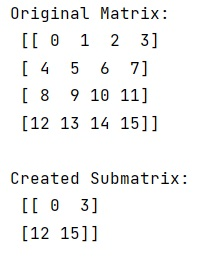# NumPy: Extract Submatrix

Given a NumPy matrix, we have to extract a submatrix from it.
Submitted by Pranit Sharma, on January 23, 2023

NumPy is an abbreviated form of Numerical Python. It is used for different types of scientific operations in python. Numpy is a vast library in python which is used for almost every kind of scientific or mathematical operation. It is itself an array which is a collection of various methods and functions for processing the arrays.

## Extracting Submatrix from a NumPy Matrix

Suppose that we are given a defined row and column structured matrix and we need to find a way to extract a submatrix from this matrix.

A matrix that contains certain rows and columns of a larger matrix is known as a submatrix.

To extract a submatrix, we will use numpy.ix_() method. This method constructs an open mesh from multiple sequences.

This function takes N 1-D sequences and returns N outputs with N dimensions each, such that the shape is 1 in all but one dimension, and the dimension with the non-unit shape value cycles through all N dimensions.

Let us understand with the help of an example,

## Python code to extract submatrix from a NumPy matrix

```# Import numpy
import numpy as np

# Creating a numpy array
arr = np.arange(16).reshape(4,4)

# Display original array
print("Original Matrix:\n",arr,"\n")

# Creating a submatrix
res = arr[np.ix_([0,3],[0,3])]

# Display result
print("Created Submatrix:\n",res,"\n")
```

Output:What's New (MCQs)

Top Interview Coding Problems/Challenges!

IncludeHelp's Blogs

Languages: » C » C++ » C++ STL » Java » Data Structure » C#.Net » Android » Kotlin » SQL
Web Technologies: » PHP » Python » JavaScript » CSS » Ajax » Node.js » Web programming/HTML
Solved programs: » C » C++ » DS » Java » C#
Aptitude que. & ans.: » C » C++ » Java » DBMS
Interview que. & ans.: » C » Embedded C » Java » SEO » HR
CS Subjects: » CS Basics » O.S. » Networks » DBMS » Embedded Systems » Cloud Computing
» Machine learning » CS Organizations » Linux » DOS
More: » Articles » Puzzles » News/Updates